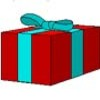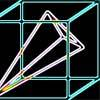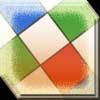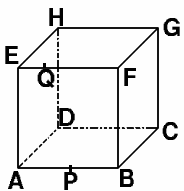#### You may also likeA box of size a cm by b cm by c cm is to be wrapped with a square piece of wrapping paper. Without cutting the paper what is the smallest square this can be?### Four Points on a Cube

What is the surface area of the tetrahedron with one vertex at O the vertex of a unit cube and the other vertices at the centres of the faces of the cube not containing O?### Instant Insanity

We have a set of four very innocent-looking cubes - each face coloured red, blue, green or white - and they have to be arranged in a row so that all of the four colours appear on each of the four long sides of the resulting cuboid.

# Plane to See

##### Age 16 to 18Challenge Level

In the cube illustrated the point $P$ is the midpoint of $AB$ and the point $Q$ is one quarter of the way along the edge $EF$.

The plane through $PDQ$ cuts the cube into two. Find the ratio of the volumes of the two pieces.